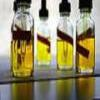#### You may also like### Mathematical Issues for Chemists

A brief outline of the mathematical issues faced by chemistry students.### Reaction Rates

Explore the possibilities for reaction rates versus concentrations with this non-linear differential equation### Catalyse That!

Can you work out how to produce the right amount of chemical in a temperature-dependent reaction?

# Extreme Dissociation

### Why do this problem?

This problem provides a good exercise in linking two areas of mathematics: concentration and logarithms. Students will need to flip between the two descriptions of acidity which will require careful, clear thinking. The context is fascinating and this will encourage a 'concrete' and practical engagement with logarithms. It will also help to give some numerical intuition as to the concentrations involved in pH calculations.

### Possible approach

This question uses the advanced concept of logarithms but will also use basic ideas about concentrations and moles. Students will need to remember basic ideas about the meaning of a 'concentration' in order to work out the extreme concentrations given in the problem.

### Key questions

What equations do you need to flip between the pH and the concentration of $H^+$ ions?
What knowledge of physical chemistry is required to do this problem?

### Possible extension

Try the final question in the sequence: Blood Buffers

### Possible support

You might try and get started using the pH formula with the problem Temperature pH